# Exactly solvable model of memristive circuits: Lyapunov functional and mean field theory

Submitted to European Physical Journal B (2017)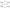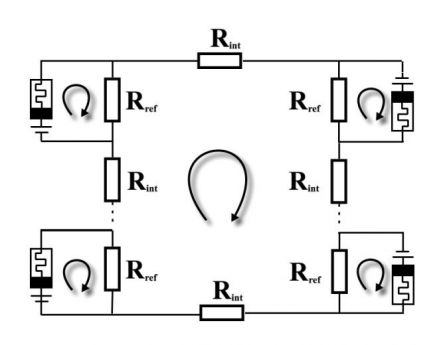In this paper we sketch a general methodology for studying the phase diagram of memristive circuits.

We construct an exactly solvable circuit of interacting memristors and study its dynamics and fixed points. This simple circuit model interpolates between decoupled circuits of isolated memristors, and memristors in series for which exact fixed points can be obtained. We introduce a Lyapunov functional that is found to be minimized along the non-equilibrium dynamics and which resembles a long-range Ising model with non-linear self-interactions. We use the Lyapunov function as an Hamiltonian to calculate, in the mean field theory approximation, the average asymptotic behavior of the circuit given a random initialization, yielding exact predictions for the case of decay to the lower resistance state, and reasonable predictions for the case of a decay to the higher resistance state.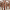#### Imaginary replica analysis of loopy regular random graphs

F. Lopez, T. Coolen

Sub. to Journal of Physics A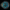#### Taming complexity

M. Reeves, S. Levin, T. Fink, A. Levina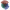#### The mathematical structure of innovation

T. Fink, I. Teimouri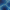#### Replica analysis of Bayesian data clustering

A. Mozeika,, A. Coolen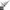#### Recursively divisible numbers

T. Fink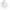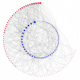#### Degree-correlations in a bursting dynamic network model

F. Vanni, P. Barucca

Journal of Economic Interaction and Coordination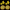#### Phase transition creates the geometry of the continuum from discrete space

R. Farr, T. Fink

Physical Review E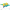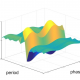#### Intelligently chosen interventions have potential to outperform the diode bridge in power conditioning

F. Liu, Y. Zhang, O. Dahlsten, F. Wang

Scientific Reports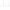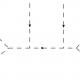#### Scale of non-locality for a system of n particles

S. Talaganis, I. Teimouri

Sub. to Physical Review D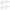#### How much can we influence the rate of innovation?

T. Fink, M. Reeves